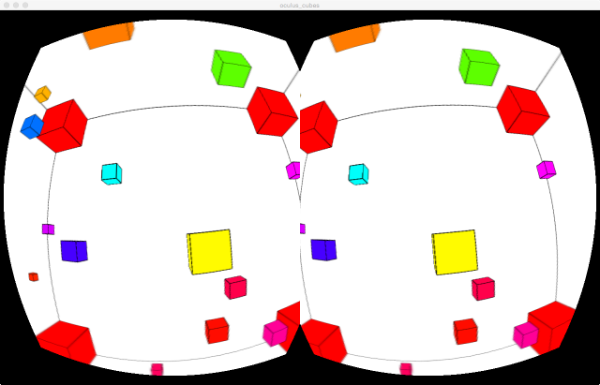## oculus experiment 2 - cubes

This is a second processing sketch I made for the oculus rift using processing and the SimpleOculusRift library. You are In the middle of a big cubical world where lots of little cubes bounce off the walls. I also implemented a invisible sphere around the viewer where the boxes bounce off, because it feels really really odd if an object is flying through you.Here is the code of the procesing sketch. To run it you need processing and the SimpleOculusRift library from the link above

``````import SimpleOculusRift.*;
int cc = 40;
boolean move = true;
SimpleOculusRift o;
PVector[] pos = new PVector[cc];
PVector[] dir = new PVector[cc];
float [] sizes = new float[cc];
color [] colors = new color[cc];

void setup() {
size(1280, 800, OPENGL);
colorMode(HSB);
o=new SimpleOculusRift( this, SimpleOculusRift.RenderQuality_Middle );
o.setBknColor(255, 255, 255);

for (int i=0; i<cc; i++) {
pos[i] = new PVector( random(6)-3, random(6)-3, random(6)-3);
if ( abs(pos[i].x) < .5 ) pos[i].x = 1;
if ( abs(pos[i].y) < .5 ) pos[i].y = 1;
if ( abs(pos[i].z) < .5 ) pos[i].z = 1;

dir[i] = new PVector( random(.1)-.05, random(.1)-.05, random(.1)-.05);

sizes[i] = random(.4)+.1;
colors[i] = color( random(255), 255, 255 );
}
strokeWeight(1);
}

void draw() {
o.draw();
if ( move ) {
for ( int i=0; i<cc; i++) {

if ( abs(pos[i].x) > 3 ) dir[i].x *= -1;
if ( abs(pos[i].y) > 3 ) dir[i].y *= -1;
if ( abs(pos[i].z) > 3 ) dir[i].z *= -1;

if ( abs(pos[i].mag()) < .5 ) {
dir[i].x *= -1;
dir[i].y *= -1;
dir[i].z *= -1;
}
}
}
}

void onDrawScene(int eye) {
noFill();
pushMatrix();
box(7);

popMatrix();

mkbox( -3, -3, -3, 1 );
mkbox( -3, 3, -3, 1 );
mkbox( 3, 3, -3, 1 );
mkbox( 3, -3, -3, 1 );

mkbox( -3, -3, 3, 1 );
mkbox( -3, 3, 3, 1 );
mkbox( 3, 3, 3, 1 );
mkbox( 3, -3, 3, 1 );

for ( int i=0; i<cc; i++) {
mkbox( pos[i].x, pos[i].y, pos[i].z, sizes[i], colors[i] );
}
}

void mkbox( float x, float y, float z, float s) {
mkbox( x, y, z, s, color(0, 255, 255));
}

void mkbox( float x, float y, float z, float s, color c ) {
stroke(0);
fill(c);
pushMatrix();
translate(x, y, z);
box(s);
popMatrix();
}

void keyPressed() {
if ( key == ' ' ) {
move = !move;
}
else {
o.resetOrientation();
}
}
``````

Leave a response

1. Hi Guru,

That's intriguing.

btw You could have a 'Ghost' or 'Spirit' -mode where you could switch on/off the odd flying through you option, either to be involved or just to observe. Who knows, like maybe in real life, perhaps there are now some creatures staring at us right in front of your faces or all around us with an OculusRift on []-) flying along with us through space ... having a cup of coffee or tea ...

Cheers,

2. Hi Guru,

I tried your cube sketch with the oculus rift DK2. It did not work properly. Is it possible to use the SimpleOculusRift library with the DK2 rift? Have you any experiences or can you recommend an other processing library for the rift?

Sincerely Marco

3. Hi, I have currently no DK2, but I as far as I know the SDK should work with newer editions too. You can try to run the example programs that are provided with the SimpleRift package or try compile a new version if that doesn´t work